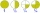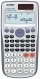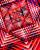# Two numbers and its product

The product of two numbers are 2/3. If on of them is 1/10, what is the other?

x =  6.6667

### Step-by-step explanation:Did you find an error or inaccuracy? Feel free to write us. Thank you!Tips to related online calculators
Need help to calculate sum, simplify or multiply fractions? Try our fraction calculator.

## Related math problems and questions:

• Evaluate expr with 2 varsIf a=6 and x=2, find the value of (2ax + 7x- 10) /(4ax-3a-2)
• Product increasedWhen the product of 2/3 and 6/10 is increased by 2/5, the result is?
• Quotient and productIf the quotient of [8/5 divided by 8/10] is added to the product of [8/14 x 7/12 x 3/8], what is the sum?
• The expressionWhat is the value of the expression ((62+60))/(23)
• Without 2Without multipying, tell whether the product 0.644 x 0.25 will be greater than 1 or less than 1? Explane how you know. Then find the product.
• The product 3The product of the two numbers is 15 ⁵/₆. If one of the numbers is 5 ²/₃, find the other.
• The product 2The product of two functions is 10. If one of them is 2 1/3, find the other one.
• The length 6The length of 12 pipes is 10 1/2 meters. (1) find the length of one pipe (2) also find the length of 7 pipes
• Divide 11Divide the product of 4 and 5/8 by 1 1/2. Write your answer as a mixed number.
• Even/odd numbersWhat is the product of 0.97 and the next odd decimal number?
• TheaterThe theater has in each row with 19 seats. Ticket to the first 10 rows is for 30 USD. In the next rows is for 15 USD. The theater was completely sold out. Revenue was 12255 USD. How many rows are in the theater?
• What is 11What is the quotient of Three-fifths and 1 Over 10?
• Quotient 3If the quotient of 8/13 and 2 is subtracted from the product of 1 3/4 and 8/21, what is the difference?
• FractionsSort fractions z1 = (6)/(11); z2 = (10)/(21); z3 = (19)/(22) by its size. Result write as three serial numbers 1,2,3.
• Expression plus minusEvaluate expression: (-1)2 . 12 – 6 : 3 + (-3) . (-2) + 22 – (-3) . 2
• Distributive propertyVerify the distributive property a×(b+c)=(a×b)+(a*c) for the rational number a=5/8, b=7/4 and c=2/3
• Simple sequenceContinue with this series of numbers: 1792,448, 112, _, _